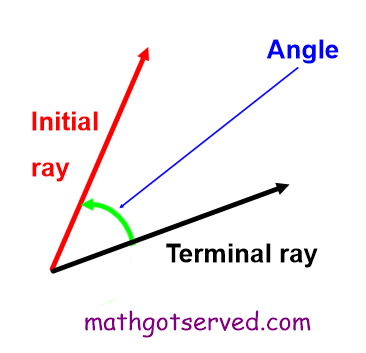MATHGOTSERVEDAngles
What is a Reference Angle?
Definition:The reference angle is the angular seperation between the terminal side and the nearest x axis. The reference angle ranges from 0 to 90 degrees.
What is an Angle?
Definition: An angle is the inclination or turn from one ray (initial) to another ray (terminal) relative to a common point.What are Coterminal Angles?
Definition: Coterminal angles are angles that have thesame terminal side. Coterminal angles are determined by adding and subtracting 360 degrees